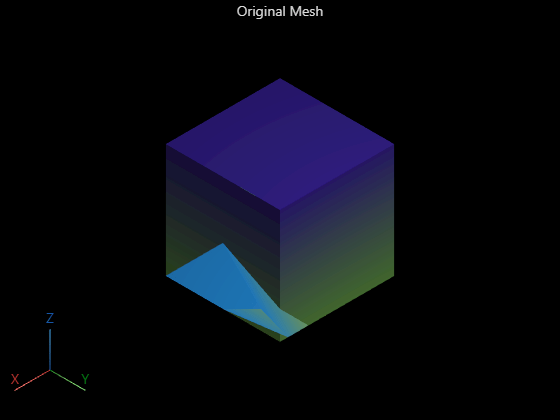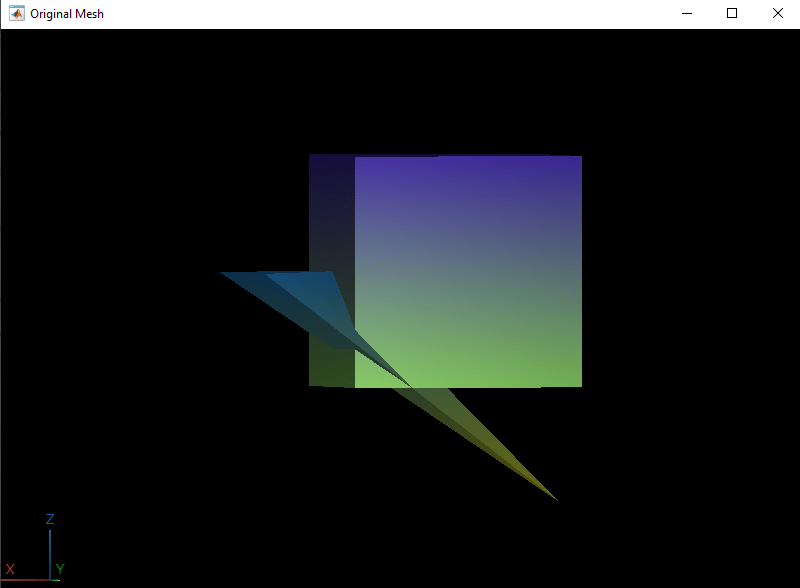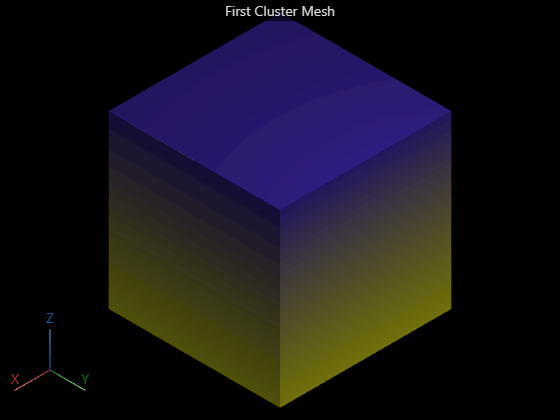# clusterConnectedFaces

Cluster connected faces

Since R2023a

## Syntax

``[faceClusterIdx,clusterNumFaces,clusterArea] = clusterConnectedFaces(surfaceMeshIn)``

## Description

example

````[faceClusterIdx,clusterNumFaces,clusterArea] = clusterConnectedFaces(surfaceMeshIn)` clusters the connected faces in the surface mesh `surfaceMeshIn`, and returns the cluster index for each face `faceClusterIdx`, the number of connected faces in each cluster `clusterNumFaces`, and the surface area of each cluster `clusterArea`. ```

## Examples

collapse all

Define 12 mesh vertices in a 12-by-3 matrix `vertices`. Each row of `vertices` specifies the `[x y z]` coordinates of a vertex. Each vertex has a vertex ID equal to its row number in `vertices`.

```vertices = [1 -1 1; 1 1 1; -1 1 1; -1 -1 1; 1 -1 -1; 1 1 -1; -1 1 -1; -1 -1 -1; 2 0 0; 2 2 0; 1 0 0; -1 0 -2];```

Use the vertices to define triangular mesh faces in the matrix `faces`. Each row of the matrix is in the form `[V1 V2 V3]`, specifying the vertex IDs of the vertices that define the triangular face.

```faces = [6 2 1; 1 5 6; 8 4 3; 3 7 8; 6 7 3; 3 2 6; 5 1 4; 4 8 5; 4 1 2; 2 3 4; 7 6 5; 5 8 7; 9 10 11; 9 10 12; 9 11 12; 10 11 12];```

Create a surface mesh from the vertices and faces.

`mesh = surfaceMesh(vertices,faces);`

Cluster the connected triangular faces of the mesh.

`[faceClusterIdx,clusterNumFaces,clusterArea] = clusterConnectedFaces(mesh);`

Visualize the surface mesh.

`surfaceMeshShow(mesh,Title="Original Mesh")`Extract the first cluster of the surface mesh.

```idx = 1:size(vertices); firstFaces = faces(faceClusterIdx==1,:); idx(unique(firstFaces))=[]; firstMesh = surfaceMesh(vertices,firstFaces); removeVertices(firstMesh,idx)```

Visualize the first cluster of the surface mesh.

`surfaceMeshShow(firstMesh,Title="First Cluster Mesh")`Extract the second cluster of the surface mesh.

```idx = 1:size(vertices); secondFaces = faces(faceClusterIdx==2,:); idx(unique(secondFaces))=[]; secondMesh = surfaceMesh(vertices,secondFaces); removeVertices(secondMesh,idx)```

Visualize the second cluster of the surface mesh.

`surfaceMeshShow(secondMesh,Title="Second Cluster Mesh")`## Input Arguments

collapse all

Input surface mesh, specified as a `surfaceMesh` object.

## Output Arguments

collapse all

Cluster indices of faces, returned as a numeric vector. Each element of the vector specifies the cluster index of a face in the surface mesh. The length of the `faceClusterIdx` vector is equal to the number of faces in `surfaceMeshIn`.

Data Types: `uint64`

Number of connected faces in each cluster, returned as a numeric vector. Each element of the vector specifies the number of connected faces in a cluster. The length of the `clusterNumFaces` vector is equal to the number of clusters created from the connected faces of `surfaceMeshIn`.

Data Types: `uint64`

Surface area of clusters, returned as a numeric vector. Each element of the vector specifies the surface area of a cluster of connected faces. The length of the `clusterArea` vector is equal to the number of clusters created from the connected faces of `surfaceMeshIn`.

Data Types: `double`

## Version History

Introduced in R2023a